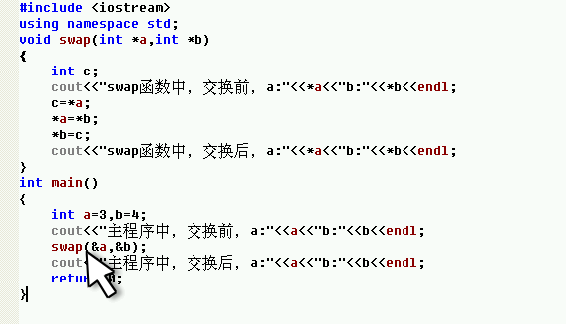C++引用中按址传递的疑问void swap（int*a，int*b）和swap(&a,&b)，*a保存a的地址嘛，为什么反而是a：<<a

5个回答

``````#include <iostream>

void swap(int *a, int *b)
{
int c;
std::cout <<"[swap][before] a=" << *a << ", b=" << *b <<std::endl;
c = *a;
*a = *b;
*b = c;
std::cout <<"[swap][after] a=" << *a << ", b=" << *b <<std::endl;
}

int main()
{
int num1=3, num2=4;
std::cout <<"[main][before] num1=" << num1 << ", num2=" << num2 <<std::endl;
swap(&num1, &num2);
std::cout <<"[main][after] num1=" << num1 << ", num2=" << num2 <<std::endl;
return 0;
}
``````

a, b是 int *类型, a和b是地址，*a和*b是值
num1和num2是 int类型，&num1和&num2是地址，num1和num2是值hhh_lijun 回复hhh_liju *a保存的就是num1的值了，是不是这样子
4 年多之前 回复hhh_lijun 指向a指针的指针，*a保存的就是num
4 年多之前 回复hhh_lijun 大神，是不是在swap函数中定义int*a，就是用int* 去定义a是int型的指针，a就是指针了，然后a指针指向num1,保存main函数传递过来的&num1，swap函数中*a就是
4 年多之前 回复

void swap（int*a，int*b）中的*只是用来标识a是int型的指针变量，指针变量接收的是int型变量a的地址。你可以把void swap（int*a，int*b）换成void swap（int*p1，int*p2）这样就好理解了。main函数中的局部变量a,b和void swap（int*a，int*b）中的a,b没有多大关系，只是存放的main函数中的局部变量a,b对应内存空间的地址。

`````` a才是地址，*a是值，*是根据地址求值，&是根据值求地址。
``````